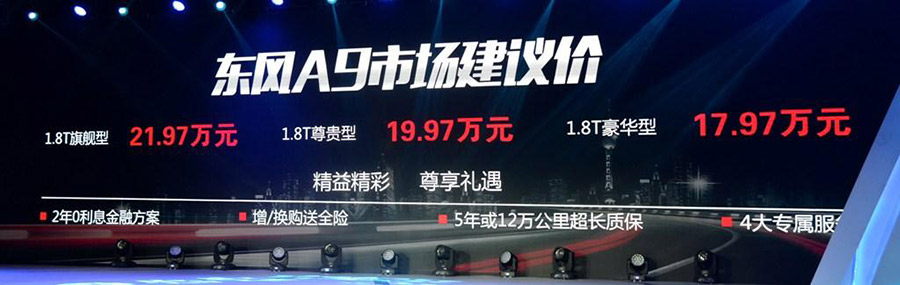# 东风风神 东风A9

## 东风A9正式上市 售17.97-21.97万元

2016年04月12日 10:50 来源：www.chextx.com 超过：9144次关注

4月12日，东风乘用车官方宣布，旗下全新中大型轿车A9正式上市。东风A9是东风乘用车体系的旗舰轿车，搭载1.8T发动机，共有3个配置车型可选，售价区间为17.97-21.97万元，详细售价请见下表：

 东风A9 市场指导售价车型售价 车型 售价（万元） 1.8T 豪华型 17.97 1.8T 尊贵型 19.97 1.8T 旗舰型 21.97东风A9是目前东风乘用车产品线中的旗舰轿车，车名中的“A”代表AEOLUS，是希腊神话风神的首字母，而“9”则是自然数的最大值，代表着顶级的含义。由于东风已经入股PSA，这款车其实是基于雪铁龙新一代C6使用的EMP2平台进行打造的。

外观方面，东风A9的整体外观设计十分大气，新车采用了“乾卦”元素的家族式设计。细节方面，新车两侧大灯和格栅相连，大灯内部还采用了LED光 源。在侧面，东风A9的线条设计比较沉稳，其还采用了大气的轮圈造型。而尾部新车设计也和车头呼应，LED光源点亮效果不错。

内饰设计方面，东风A9借鉴了标致408的设计，不过整体风格更有商务范儿。细节方面，该车使用了大面积的木纹装饰，在中控台中配备了石英表和大尺寸的液晶显示屏，这些都让车内看上去更上档次。

东风A9的长宽高依次为5066mm/1858mm/1470mm，轴距达到2900mm，定位在中大型车级别。乘坐空间方面，176cm身高的体验者在后排有着两拳多的腿部空间，空间表现符合同级别表现。

东风A9的定位是行政级座驾，在配置方面自然也是非常丰富，除了像一键启动、电子手刹、360°影像这些目前较为主流的配置外，还采用了 Nappa真皮、带按摩/加热/通风功能的前后排座椅、12个扬声器的Infinity环绕音响、空气净化系统、HUD抬头显示、四区独立空调等。

动力方面，东风A9搭载的是一台1.8T发动机，这款发动机的最大功率为204马力，峰值扭矩为280牛·米。在传动系统部分，这款车匹配的是6挡手自一体变速箱，带有运动模式和雪地模式可选。

东风A9的前身其实就是东风1号车型，东风1号概念车于2014年北京车展首发，而量产车型在2015年上海车展正式发布。又经过了近1年的不断调试，在2016年的2月底，这款车正式和媒体见面并定名为东风A9。

纵观目前中国品牌中大型车市场，这个市场的产品并不是很多，上市销售的仅有众泰Z700、红旗H7以及绅宝D80三款，还有即将上市的传祺 GA8。向上走一直是中国品牌在不断追求的梦想，而目前几款敢于尝试的产品，我们也都是应该鼓励的。如果说存在竞争关系的话，编辑认为中国品牌的这几款车 其实竞争关系不大，他们的主要对手其实还是那些价位接近的例如君越、帕萨特等合资品牌中型车。而中国品牌中大型车在市场中的优势是空间和配置上，而不足则 还是品牌和一些制造工艺。

2015年，东风风神正在逐步完善3系（A30）、6系（A60、L60）、9系(东风A9)以及X系（AX7等SUV）的产品线，未来将采用 “轿车+SUV”并进的路线。而在2016年，东风乘用车设定了销量12.5万辆的目标，更多新产品的推出，也将助力东风乘用车实现自己的规划。

#### 相关文章

0-500 字已有评论 0条 查看评论>>

### 东风A9### 最新资讯

﻿
• 快速找车
• 选择品牌
• 选择品牌
• A  奥迪
• A  阿斯顿·马丁
• A  阿尔法·罗密欧
• B  宝沃
• B  布加迪
• B  巴博斯
• B  保时捷
• B  宾利
• B  奔驰
• B  宝马
• B  本田
• B  别克
• B  标致
• B  比亚迪
• B  宝骏
• B  北汽制造
• B  北汽新能源
• B  北汽幻速
• B  北汽威旺
• B  北京汽车
• B  奔腾
• B  北汽绅宝
• C  长安
• C  长安商用
• C  长城
• C  昌河
• D  大众
• D  道奇
• D  DS
• D  东南
• D  东风风神
• D  东风风行
• D  东风小康
• D  东风风度
• D  东风
• F  福特
• F  丰田
• F  菲亚特
• F  法拉利
• F  福田
• F  福迪
• F  福汽启腾
• G  观致
• G  广汽传祺
• G  广汽吉奥
• G  GMC
• H  红旗
• H  汉腾汽车
• H  哈弗
• H  哈飞
• H  海格
• H  海马
• H  华颂
• H  黄海
• H  华泰
• H  恒天
• J  吉利汽车
• J  捷豹
• J  Jeep
• J  江淮
• J  江铃
• J  金杯
• J  九龙
• J  金旅
• K  凯翼
• K  凯迪拉克
• K  克莱斯勒
• K  科尼塞克
• K  卡威
• K  开瑞
• L  路虎
• L  林肯
• L  劳斯莱斯
• L  兰博基尼
• L  雷克萨斯
• L  铃木
• L  雷诺
• L  理念
• L  力帆
• L  莲花汽车
• L  猎豹
• L  路特斯
• L  陆风
• M  马自达
• M  MG
• M  MINI
• M  玛莎拉蒂
• M  摩根
• M  迈凯轮
• N  纳智捷
• O  欧宝
• O  讴歌
• O  欧朗
• Q  奇瑞
• Q  起亚
• Q  启辰
• R  日产
• R  荣威
• R  瑞麒
• S  三菱
• S  斯威汽车
• S  萨博
• S  smart
• S  斯柯达
• S  斯巴鲁
• S  思铭
• S  双龙
• S  上汽大通
• S  双环
• T  特斯拉
• T  腾势
• W  沃尔沃
• W  五菱汽车
• W  五十铃
• W  威兹曼
• W  威麟
• X  现代
• X  雪佛兰
• X  雪铁龙
• X  西雅特
• Y  一汽
• Y  英菲尼迪
• Y  英致
• Y  依维柯
• Y  野马汽车
• Y  永源
• Z  众泰
• Z  中华
• Z  中兴
• Z  知豆
• 选择车系
• 选择车系
• 车型对比
• 选择品牌
• 选择品牌
• A  奥迪
• A  阿斯顿·马丁
• A  阿尔法·罗密欧
• B  宝沃
• B  布加迪
• B  巴博斯
• B  保时捷
• B  宾利
• B  奔驰
• B  宝马
• B  本田
• B  别克
• B  标致
• B  比亚迪
• B  宝骏
• B  北汽制造
• B  北汽新能源
• B  北汽幻速
• B  北汽威旺
• B  北京汽车
• B  奔腾
• B  北汽绅宝
• C  长安
• C  长安商用
• C  长城
• C  昌河
• D  大众
• D  道奇
• D  DS
• D  东南
• D  东风风神
• D  东风风行
• D  东风小康
• D  东风风度
• D  东风
• F  福特
• F  丰田
• F  菲亚特
• F  法拉利
• F  福田
• F  福迪
• F  福汽启腾
• G  观致
• G  广汽传祺
• G  广汽吉奥
• G  GMC
• H  红旗
• H  汉腾汽车
• H  哈弗
• H  哈飞
• H  海格
• H  海马
• H  华颂
• H  黄海
• H  华泰
• H  恒天
• J  吉利汽车
• J  捷豹
• J  Jeep
• J  江淮
• J  江铃
• J  金杯
• J  九龙
• J  金旅
• K  凯翼
• K  凯迪拉克
• K  克莱斯勒
• K  科尼塞克
• K  卡威
• K  开瑞
• L  路虎
• L  林肯
• L  劳斯莱斯
• L  兰博基尼
• L  雷克萨斯
• L  铃木
• L  雷诺
• L  理念
• L  力帆
• L  莲花汽车
• L  猎豹
• L  路特斯
• L  陆风
• M  马自达
• M  MG
• M  MINI
• M  玛莎拉蒂
• M  摩根
• M  迈凯轮
• N  纳智捷
• O  欧宝
• O  讴歌
• O  欧朗
• Q  奇瑞
• Q  起亚
• Q  启辰
• R  日产
• R  荣威
• R  瑞麒
• S  三菱
• S  斯威汽车
• S  萨博
• S  smart
• S  斯柯达
• S  斯巴鲁
• S  思铭
• S  双龙
• S  上汽大通
• S  双环
• T  特斯拉
• T  腾势
• W  沃尔沃
• W  五菱汽车
• W  五十铃
• W  威兹曼
• W  威麟
• X  现代
• X  雪佛兰
• X  雪铁龙
• X  西雅特
• Y  一汽
• Y  英菲尼迪
• Y  英致
• Y  依维柯
• Y  野马汽车
• Y  永源
• Z  众泰
• Z  中华
• Z  中兴
• Z  知豆
• 选择车系
• 选择车系
• 选择车型
• 选择车型
• 意见反馈